# Lions Weekly: May 11, 2022# A Note From Mr. Sandefur

Lion Family,

With all that is going on throughout our town, state, nation, and the world, I would be negligent if I didn’t mention our responsibility as the body of Christ to love and welcome every single person that is a part of our community. It is our deepest desire that TKA would be an earthly representation of our heavenly destination, being a community that is united in the love of Christ regardless of background, ethnicity, socio-economic class, or anything else. As we train our students and faculty to be loving and welcoming to all who come on our campus, I humbly ask that all school community members do so as well. I pray that we live out these words of the Apostle Paul found in 2 Corinthians 13:11 as a school community, “Finally, brothers and sisters, rejoice! Strive for full restoration, encourage one another, be of one mind, and live in peace. And the God of love and peace will be with you.”

As I write this letter to you, I struggle with the reality that our academic year is rapidly approaching its conclusion. The next few days will be packed full of activities as we prepare to graduate the Class of 2022, honor our students for their academic achievements, conclude athletic competitions, and so much more while ensuring that our mission of developing servant leaders for Jesus Christ is the driving force of all that we do. I appreciate the partnership of every family that commits daily to investing in Christ-centered education here at The King’s Academy and look forward to seeing you at one of the many upcoming events on campus.

In Christ Alone,

Jeremy Sandefur
President

 table div table+table+table+table div table{width:100%;padding:0}table div table+table+table+table div table img{width:96.23%;padding:0;float:none}table div table+table+table+table div table td{width:100%;padding:0 1.88% 18px}/* styles */# Elementary Spring Concert

## Thursday, May 12

Join us Thursday, May 12, at 6:30 p.m. in Woody Auditorium as our Elementary Students present God's Not Dead! To view the program's order of service, click here.

 table div table+table+table+table+table+table div table{width:100%;padding:0}table div table+table+table+table+table+table div table img{width:96.23%;padding:0;float:none}table div table+table+table+table+table+table div table td{width:100%;padding:0 1.88% 18px}/* styles */# Friday, May 13

## Events

▪ Senior Breakfast at 8:00 a.m.
▪ Legacy Walk at 9:00 a.m.
▪ Big/Little Buddy End of the Year Party at 10:30 a.m.
▪ Middle School Field Day from 1:00 p.m. to 3:00 p.m. at Tibbetts and the Baseball Field
▪ High School Field Day from 1:00p.m. to 3:00p.m. at Huskey Field and Stokley Gym
 ▪ Senior Breakfast at 8:00 a.m.
 ▪ Legacy Walk at 9:00 a.m.
 ▪ Big/Little Buddy End of the Year Party at 10:30 a.m.
 ▪ Middle School Field Day from 1:00 p.m. to 3:00 p.m. at Tibbetts and the Baseball Field
 ▪ High School Field Day from 1:00p.m. to 3:00p.m. at Huskey Field and Stokley Gym
 table div table+table+table+table+table+table+table+table div table{width:100%;padding:0}table div table+table+table+table+table+table+table+table div table img{width:96.23%;padding:0;float:none}table div table+table+table+table+table+table+table+table div table td{width:100%;padding:0 1.88% 18px}/* styles */# Baccalaureate Service

You are cordially invited to join us at Seymour First Baptist on Sunday, May 15, at 3:00 p.m. as we honor the graduating class of 2022. We will honor our students through words of encouragement, biblical challenges, a time of corporate worship, and a dedicated time of prayer and commissioning for our graduates.

 table div table+table+table+table+table+table+table+table+table+table div table{width:100%;padding:0}table div table+table+table+table+table+table+table+table+table+table div table img{width:96.23%;padding:0;float:none}table div table+table+table+table+table+table+table+table+table+table div table td{width:100%;padding:0 1.88% 18px}/* styles */# Ways to help with the TKA Kenya Trip

Our team of TKA teachers will be serving the four hundred students of Empukani Primary (Kenya) in June by offering a Vacation Bible School along with teacher training. The focus of the VBS will be “I AM: The Promises of God.”

Many have asked how they could support the efforts of this trip through prayer and giving. The following items are needed and all donations are fully appreciated:

Snacks
· Vanilla wafers
· Twizzlers
· Goldfish
· Hard candy (not individually wrapped)

Crafts
· Embroidery floss (red, green, blue, yellow, and orange)
· Clear contact paper
· Colored sharpies
· White handkerchiefs (50 for \$20 on Amazon)
· Beads (black, red, green, white, yellow(gold), and blue)
· Pipe cleaners
· Crayons
· Markers
· Colored pencils
· Small sharpeners for colored pencils and crayons

We will have to pack these items and travel with them so we are looking for large sealed packages instead of individually wrapped packages. Ms. Shannon, Mrs. Smith, and Mrs. Q will be collecting these items in their classrooms; however, items can be dropped off in the High School or Elementary offices as well.

Any and all donations are truly appreciated!

Sincerely,

Kenya Mission Team

 table div table+table+table+table+table+table+table+table+table+table+table+table div table{width:100%;padding:0}table div table+table+table+table+table+table+table+table+table+table+table+table div table img{width:96.23%;padding:0;float:none}table div table+table+table+table+table+table+table+table+table+table+table+table div table td{width:100%;padding:0 1.88% 18px}/* styles */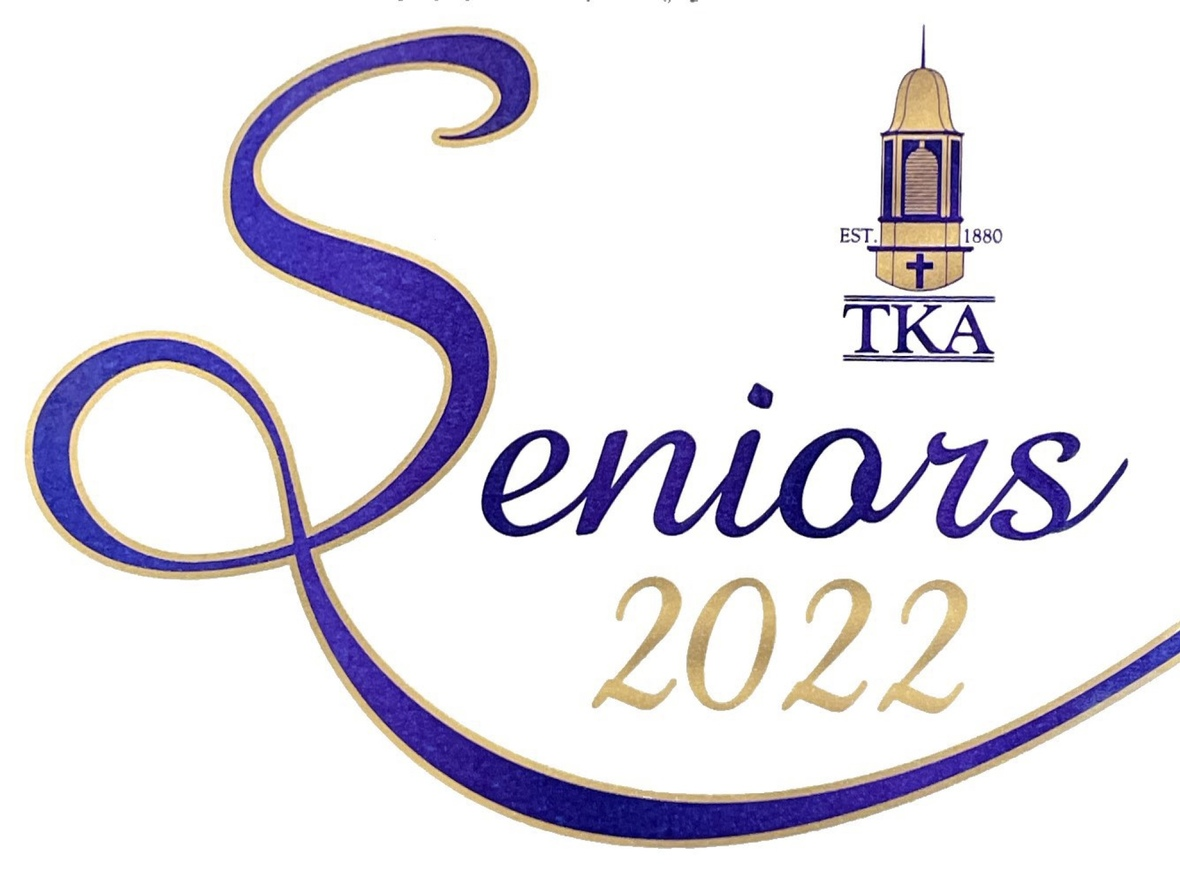On behalf of the faculty and graduating class of 2022, The King's Academy would like to invite you to the Commencement Exercises on Friday, May 20, at 7:00p.m. in Tibbetts.

 table div table+table+table+table+table+table+table+table+table+table+table+table+table+table div table{width:100%;padding:0}table div table+table+table+table+table+table+table+table+table+table+table+table+table+table div table img{width:96.23%;padding:0;float:none}table div table+table+table+table+table+table+table+table+table+table+table+table+table+table div table td{width:100%;padding:0 1.88% 18px}/* styles */# End of the Year Art Show!

Students in the art classes have done an amazing job this year and would like to share their work with the TKA community.

Artwork will be on display in the lobby by Woody Auditorium and along the main hallway. Please come and enjoy original artwork by our talented students at the following art shows:

High School Art Show:
Wednesday, May 18 through Friday, May 20
Artwork by Digital Arts students will be on display on the hallway tv monitors during this time.

Middle School Art Show: Monday, May 23 through Wednesday, May 25.

 table div table+table+table+table+table+table+table+table+table+table+table+table+table+table+table+table div table{width:100%;padding:0}table div table+table+table+table+table+table+table+table+table+table+table+table+table+table+table+table div table img{width:96.23%;padding:0;float:none}table div table+table+table+table+table+table+table+table+table+table+table+table+table+table+table+table div table td{width:100%;padding:0 1.88% 18px}/* styles */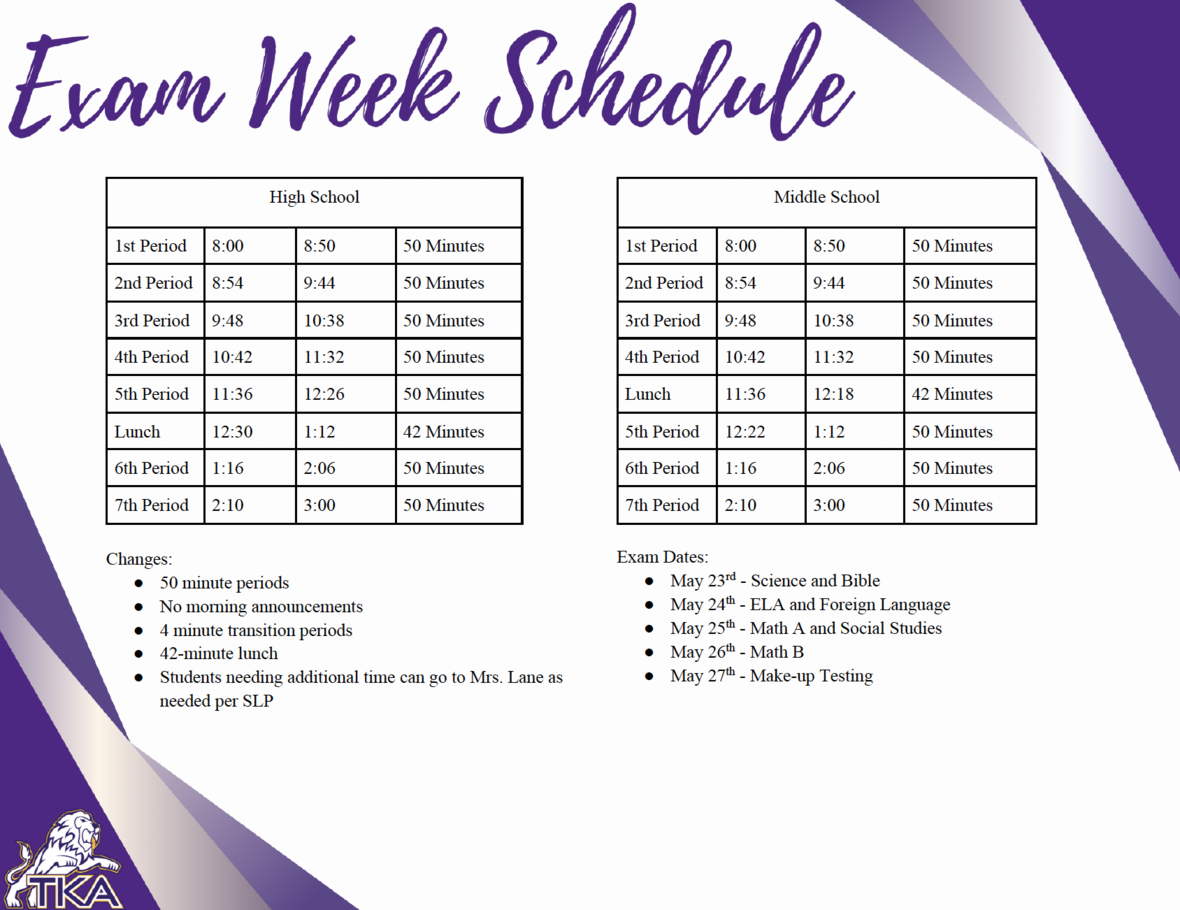table div table+table+table+table+table+table+table+table+table+table+table+table+table+table+table+table+table+table div table{width:100%;padding:0}table div table+table+table+table+table+table+table+table+table+table+table+table+table+table+table+table+table+table div table img{width:96.23%;padding:0;float:none}table div table+table+table+table+table+table+table+table+table+table+table+table+table+table+table+table+table+table div table td{width:100%;padding:0 1.88% 18px}/* styles */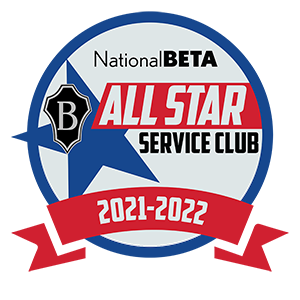# TKA's National Beta Club Wins National Service Award

The National Beta Club at The King's Academy has proudly been named a 2022 National Beta All Star Service Award winner. The club earned this award by spending an average of 50-75 hours per member serving their school, community, and state this year.

As one of the four pillars of National Beta, service is encouraged to help students learn to demonstrate the motto: Let Us Lead by Serving Others. By showing dedication to service, The King's Academy Betas helped contribute to over 1,086,625 hours served by clubs across the nation this year. Collectively, Betas also raised \$944,963 for various organizations and charities. The club at The King's Academy joins a group of only 95 other schools to earn the award this year.

Members will each receive a commemorative pin and the club will receive a plaque to proudly display in their school.

With more than 500,000 active members and 9,000 chapters nationally and internationally, National Beta has become the nation’s largest independent, non-profit, educational youth organization. National Beta recognizes outstanding achievement, promotes character and social responsibility, encourages service involvement to school and community, and fosters leadership skills. National Junior Beta includes grades 4-8 and National Senior Beta includes grades 9-12. The National Beta program offers over \$300,000 annually to its Senior Beta Members. Visit betaclub.org for more information.

 table div table+table+table+table+table+table+table+table+table+table+table+table+table+table+table+table+table+table+table+table div table{width:100%;padding:0}table div table+table+table+table+table+table+table+table+table+table+table+table+table+table+table+table+table+table+table+table div table img{width:96.23%;padding:0;float:none}table div table+table+table+table+table+table+table+table+table+table+table+table+table+table+table+table+table+table+table+table div table td{width:100%;padding:0 1.88% 18px}/* styles */# End of the Year Events

As the end of the year quickly approaches, we want to be sure and communicate any calendar events as soon as we are able. Below are upcoming events for the Month of May we would like for you to mark on your calendar.

Thursday, May 12:
- Elementary Spring Concert at 6:30 p.m. in Woody Auditorium
Friday, May 13:
- Senior Breakfast at 8:00 a.m.
- Legacy Walk at 9:00 a.m.
- Big/Little Buddy End of the Year Party at 10:30 a.m.
- Middle School Field Day from 1:00 p.m. to 3:00 p.m. at Tibbetts and the Baseball Field
- High School Field Day from 1:00p.m. to 3:00p.m. at Huskey Field and Stokley Gym
Sunday, May 15
- Baccalaureate Service at 3:00 p.m. at Seymour First Baptist
Tuesday, May 17
- Used Uniform Sale from 3:00 p.m. to 6:00 p.m. in Dr. Carroll Reece Reagan Pavilion
Thursday, May 19
- PTF Meeting at 3:15 in Brandes
Friday, May 20:
- High School Awards Day at 10:00 a.m. in Tibbetts
- Senior Graduation at 7:00 p.m. in Tibbetts
Monday, May 23:
- PreK4 Graduation at 6:00 p.m. in Woody Auditorium
Tuesday, May 24:
- HS Exams
- Sports Physicals at 3:00 p.m. in Tibbetts
- Kindergarten Graduation at 6:00 p.m. in Woody Auditorium
Wednesday, May 25
- HS Exams
Thursday, May 26:
- HS Exams
- 5th Grade promotion from 8:15 a.m. to 9:15 a.m. in Woody Auditorium
- Elementary classroom end of the year programs
Friday, May 27:
- Make-up HS Exams
- Middle School Awards at 9:00 a.m. in Woody Auditorium
- 8th Grade Promotion at 10:00 a.m. in Woody Auditorium
- Half-day dismissal at 11:30 a.m. No aftercare will be provided.

 table div table+table+table+table+table+table+table+table+table+table+table+table+table+table+table+table+table+table+table+table+table+table div table{width:100%;padding:0}table div table+table+table+table+table+table+table+table+table+table+table+table+table+table+table+table+table+table+table+table+table+table div table img{width:96.23%;padding:0;float:none}table div table+table+table+table+table+table+table+table+table+table+table+table+table+table+table+table+table+table+table+table+table+table div table td{width:100%;padding:0 1.88% 18px}/* styles */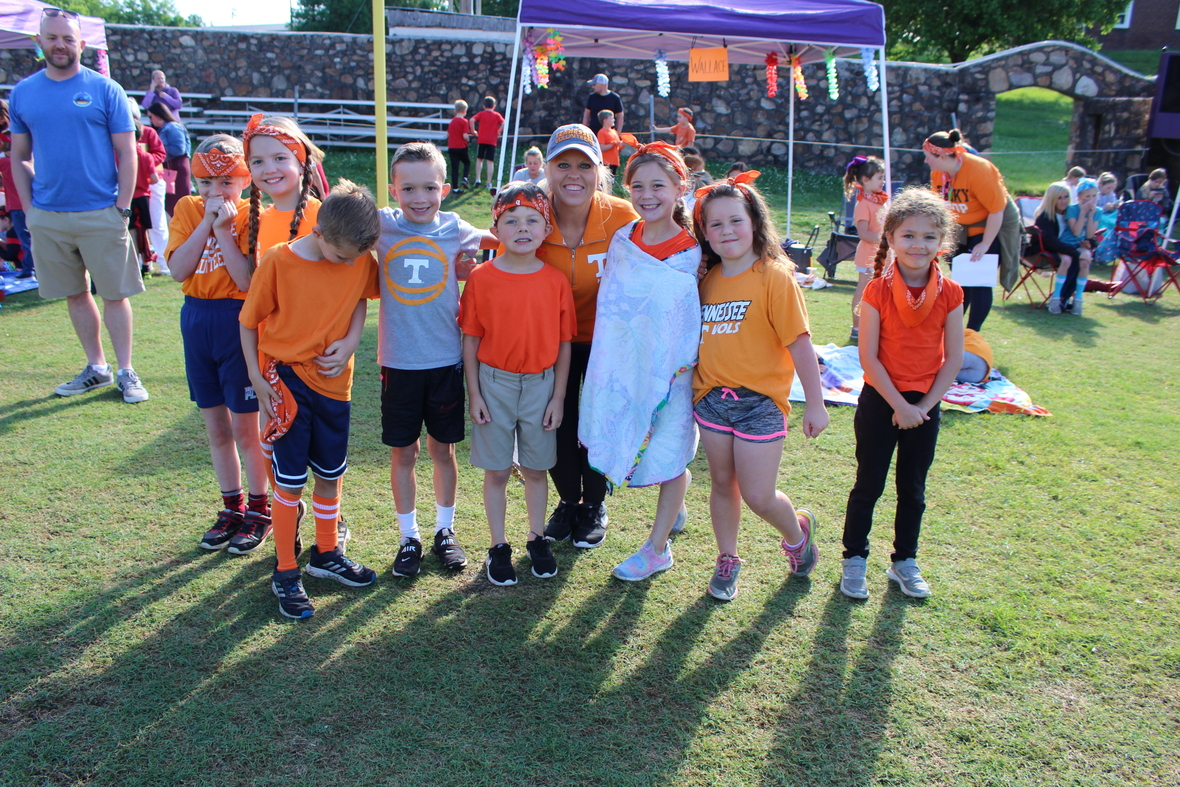# Elementary Field Day Recap

Field Day was a super successful day! We greatly appreciate all that Mrs. Robin did to prepare such a fun day of events. We want to thank our parent and MS/HS volunteers for all of their help to make field day run smoothly and successfully.

Our students had a blast and ROARed with Christlike character all throughout field day events. We love to see our little lions encouraging, supporting, and cheering for one another.

Way to ROAR little lions!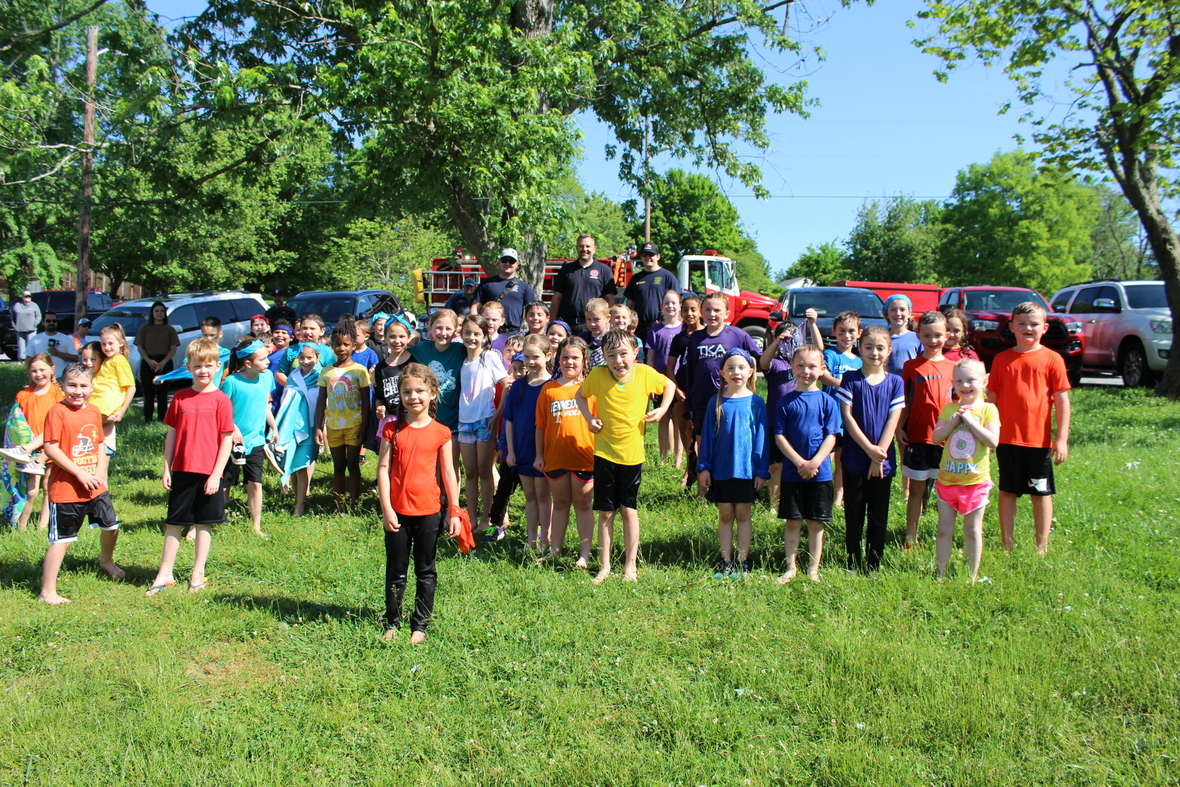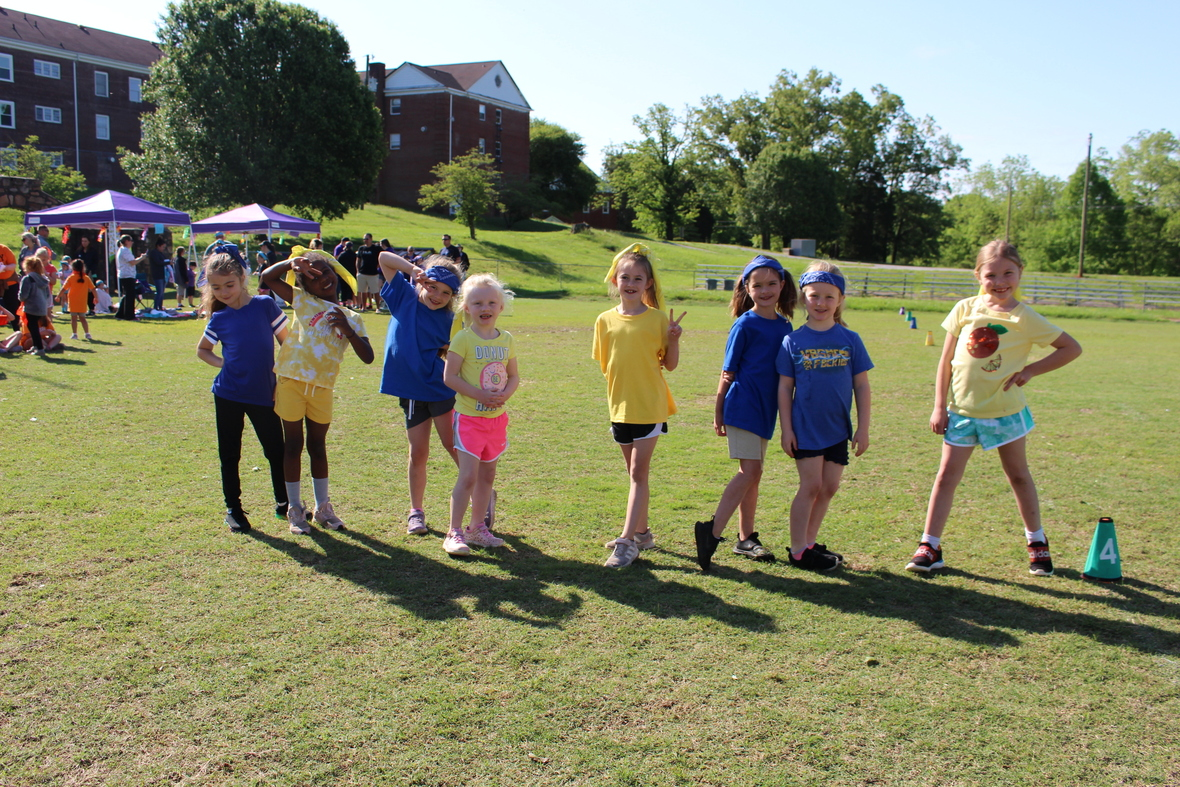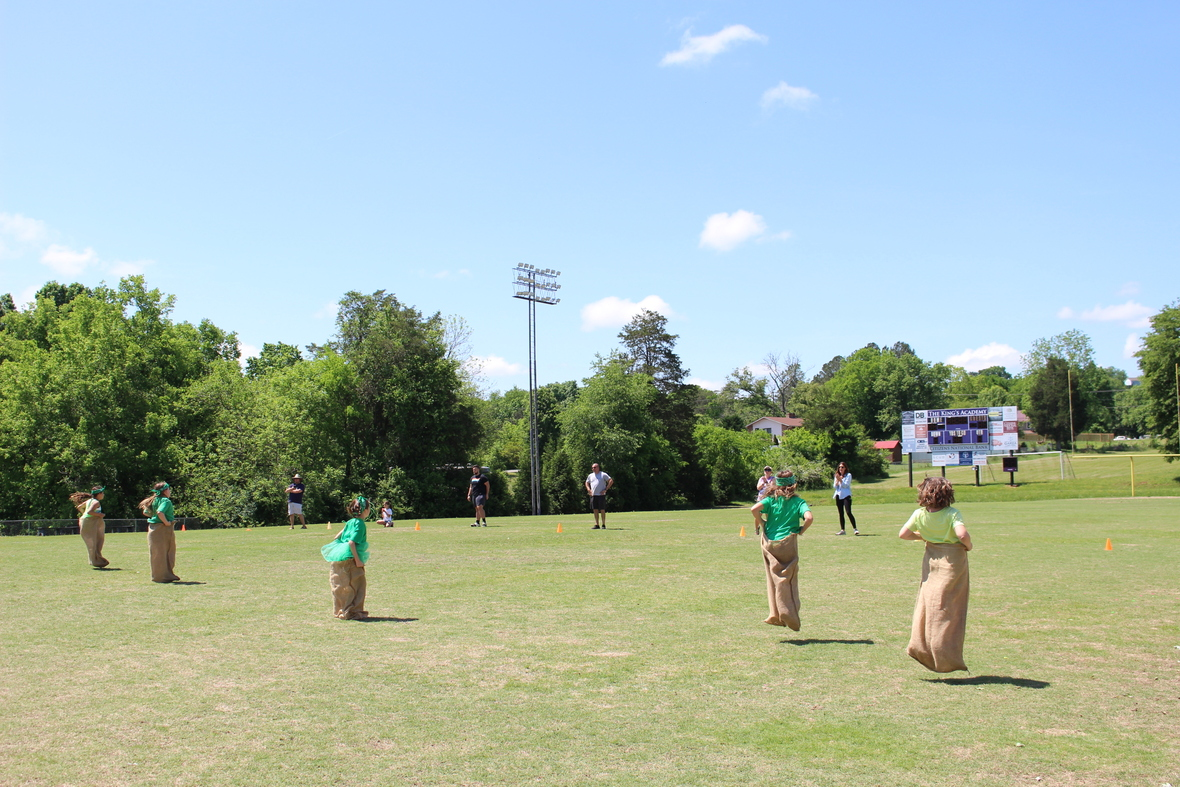table div table+table+table+table+table+table+table+table+table+table+table+table+table+table+table+table+table+table+table+table+table+table+table+table+table+table div table{width:100%;padding:0}table div table+table+table+table+table+table+table+table+table+table+table+table+table+table+table+table+table+table+table+table+table+table+table+table+table+table div table img{width:96.23%;padding:0;float:none}table div table+table+table+table+table+table+table+table+table+table+table+table+table+table+table+table+table+table+table+table+table+table+table+table+table+table div table td{width:100%;padding:0 1.88% 18px}/* styles */# Ferns for Sale

TKA Garden & Greenhouse is selling ferns! The small ferns are \$5 and large ferns are \$10.

 table div table+table+table+table+table+table+table+table+table+table+table+table+table+table+table+table+table+table+table+table+table+table+table+table+table+table+table+table div table{width:100%;padding:0}table div table+table+table+table+table+table+table+table+table+table+table+table+table+table+table+table+table+table+table+table+table+table+table+table+table+table+table+table div table img{width:96.23%;padding:0;float:none}table div table+table+table+table+table+table+table+table+table+table+table+table+table+table+table+table+table+table+table+table+table+table+table+table+table+table+table+table div table td{width:100%;padding:0 1.88% 18px}/* styles */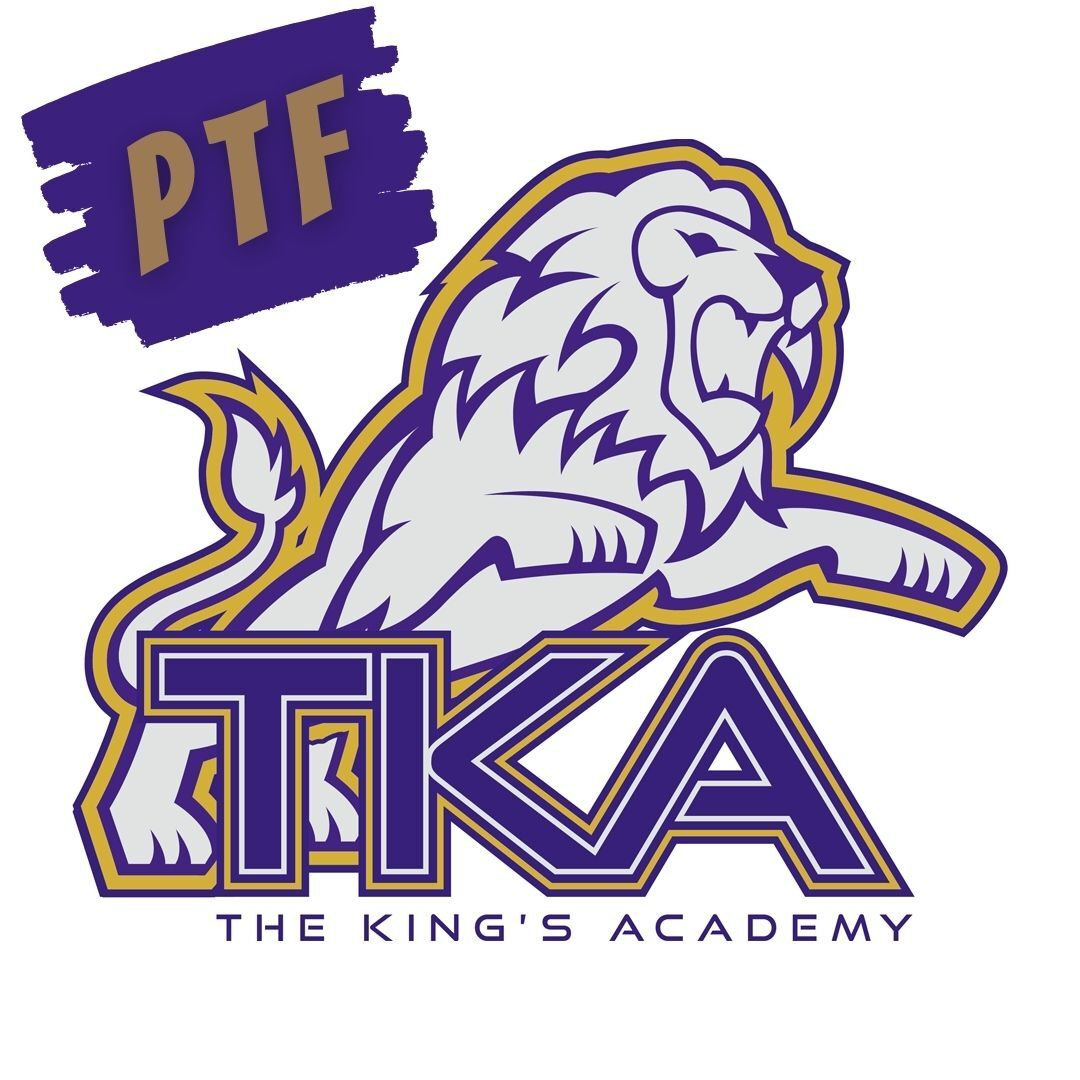# PTF

Thursday, May 19, will be the last PTF meeting of the year in Brandes from 3:15 - 4:15. All members are encouraged to attend.

 table div table+table+table+table+table+table+table+table+table+table+table+table+table+table+table+table+table+table+table+table+table+table+table+table+table+table+table+table+table+table div table{width:100%;padding:0}table div table+table+table+table+table+table+table+table+table+table+table+table+table+table+table+table+table+table+table+table+table+table+table+table+table+table+table+table+table+table div table img{width:96.23%;padding:0;float:none}table div table+table+table+table+table+table+table+table+table+table+table+table+table+table+table+table+table+table+table+table+table+table+table+table+table+table+table+table+table+table div table td{width:100%;padding:0 1.88% 18px}/* styles */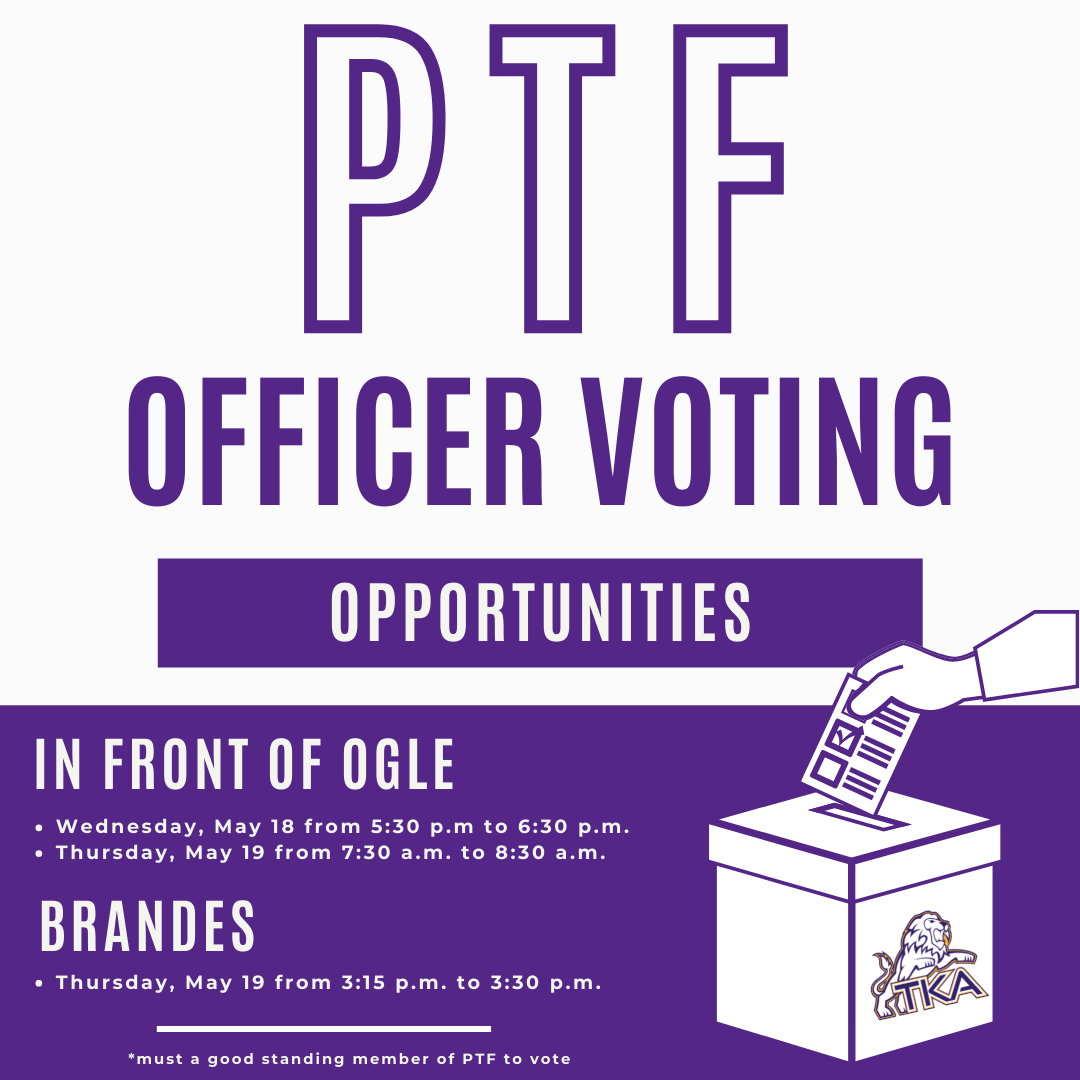# PTF Officer Voting

Thank you to all PTF members who submitted nominations for the 2022-2023 PTF Executive Board.

Voting will take place in person only, by current PTF member in good standing.

Opportunities to vote will be on the following days and times:

▪ Wednesday May 18, from 5:30 p.m. to 630 p.m. in front of Ogle
▪ Thursday May 19, from 7:30 a.m. to 8:30 a.m. in front of Ogle
▪ Thursday, May 19, from 3:15 p.m. to 3:30 p.m. in Brandes
 ▪ Wednesday May 18, from 5:30 p.m. to 630 p.m. in front of Ogle
 ▪ Thursday May 19, from 7:30 a.m. to 8:30 a.m. in front of Ogle
 ▪ Thursday, May 19, from 3:15 p.m. to 3:30 p.m. in Brandes

At 3:30 p.m. on Thursday, May 19, the votes will be tallied and new PTF Board will be announced.

 table div table+table+table+table+table+table+table+table+table+table+table+table+table+table+table+table+table+table+table+table+table+table+table+table+table+table+table+table+table+table+table+table div table{width:100%;padding:0}table div table+table+table+table+table+table+table+table+table+table+table+table+table+table+table+table+table+table+table+table+table+table+table+table+table+table+table+table+table+table+table+table div table img{width:96.23%;padding:0;float:none}table div table+table+table+table+table+table+table+table+table+table+table+table+table+table+table+table+table+table+table+table+table+table+table+table+table+table+table+table+table+table+table+table div table td{width:100%;padding:0 1.88% 18px}/* styles */# Used Uniform Sale

When: Tuesday, May 17, from 3pm-6pm

What: Bring all your outgrown, new or used polos, pants/shorts/skirts, spirit wear, shoes, backpacks/bags & ANYTHING TKA-related. Don’t forget about Daddy-Daughter & Prom dresses/attire as well as sport equipment. If TKA plays it, bring it!

Where: We will set up a “uniform shop” under the Dr. Carroll Reece Reagan Pavilion. You can set up and sell your own items for whatever price you choose.

By reusing and repurposing these items, hopefully we can all receive a blessing. Stay as little or as long as you wish!

If it is not allowed in the dress code, please do not bring it (remember that pink & light blue are not allowed starting next school year).

 table div table+table+table+table+table+table+table+table+table+table+table+table+table+table+table+table+table+table+table+table+table+table+table+table+table+table+table+table+table+table+table+table+table+table div table{width:100%;padding:0}table div table+table+table+table+table+table+table+table+table+table+table+table+table+table+table+table+table+table+table+table+table+table+table+table+table+table+table+table+table+table+table+table+table+table div table img{width:96.23%;padding:0;float:none}table div table+table+table+table+table+table+table+table+table+table+table+table+table+table+table+table+table+table+table+table+table+table+table+table+table+table+table+table+table+table+table+table+table+table div table td{width:100%;padding:0 1.88% 18px}/* styles */# Missing Keys?

A set of keys were found in the elementary parking lot. If you are missing keys, please contact Mrs. Gioioso in the elementary office at tgioioso@thekingsacademy.net.

 table div table+table+table+table+table+table+table+table+table+table+table+table+table+table+table+table+table+table+table+table+table+table+table+table+table+table+table+table+table+table+table+table+table+table+table+table div table{width:100%;padding:0}table div table+table+table+table+table+table+table+table+table+table+table+table+table+table+table+table+table+table+table+table+table+table+table+table+table+table+table+table+table+table+table+table+table+table+table+table div table img{width:96.23%;padding:0;float:none}table div table+table+table+table+table+table+table+table+table+table+table+table+table+table+table+table+table+table+table+table+table+table+table+table+table+table+table+table+table+table+table+table+table+table+table+table div table td{width:100%;padding:0 1.88% 18px}/* styles */# 27th Annual Golf Tournament

Join us at Egwani Farms on June 13, for our 27th Annual Golf Tournament to benefit TKA's football team!

Gather your company, friends or family and purchase one of the following sponsorships:
- Purple Sponsor includes a 4-person team and a hole sponsorship for \$500
- Silver Sponsor includes an individual player and a hole sponsorship for \$200
- Individual players can participate for \$100

 table div table+table+table+table+table+table+table+table+table+table+table+table+table+table+table+table+table+table+table+table+table+table+table+table+table+table+table+table+table+table+table+table+table+table+table+table+table+table div table{width:100%;padding:0}table div table+table+table+table+table+table+table+table+table+table+table+table+table+table+table+table+table+table+table+table+table+table+table+table+table+table+table+table+table+table+table+table+table+table+table+table+table+table div table img{width:96.23%;padding:0;float:none}table div table+table+table+table+table+table+table+table+table+table+table+table+table+table+table+table+table+table+table+table+table+table+table+table+table+table+table+table+table+table+table+table+table+table+table+table+table+table div table td{width:100%;padding:0 1.88% 18px}/* styles */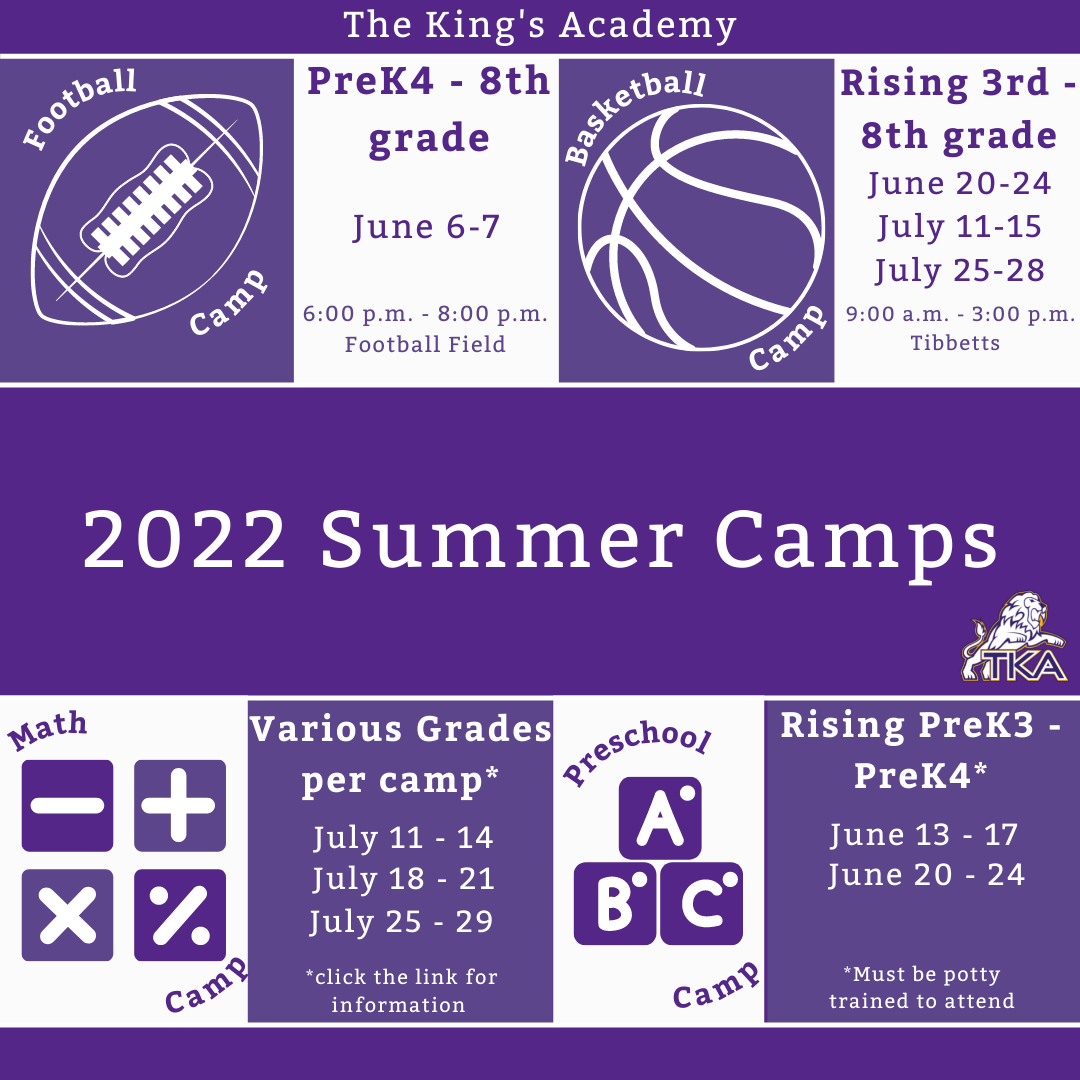# 2022 Summer Camps

We are excited to offer a variety of camps for our TKA Lions this summer! Each camp will be listed in the link below with links to register for each event. We are looking forward to seeing our Lions on campus at these fun camps!

 table div table+table+table+table+table+table+table+table+table+table+table+table+table+table+table+table+table+table+table+table+table+table+table+table+table+table+table+table+table+table+table+table+table+table+table+table+table+table+table+table div table{width:100%;padding:0}table div table+table+table+table+table+table+table+table+table+table+table+table+table+table+table+table+table+table+table+table+table+table+table+table+table+table+table+table+table+table+table+table+table+table+table+table+table+table+table+table div table img{width:96.23%;padding:0;float:none}table div table+table+table+table+table+table+table+table+table+table+table+table+table+table+table+table+table+table+table+table+table+table+table+table+table+table+table+table+table+table+table+table+table+table+table+table+table+table+table+table div table td{width:100%;padding:0 1.88% 18px}/* styles */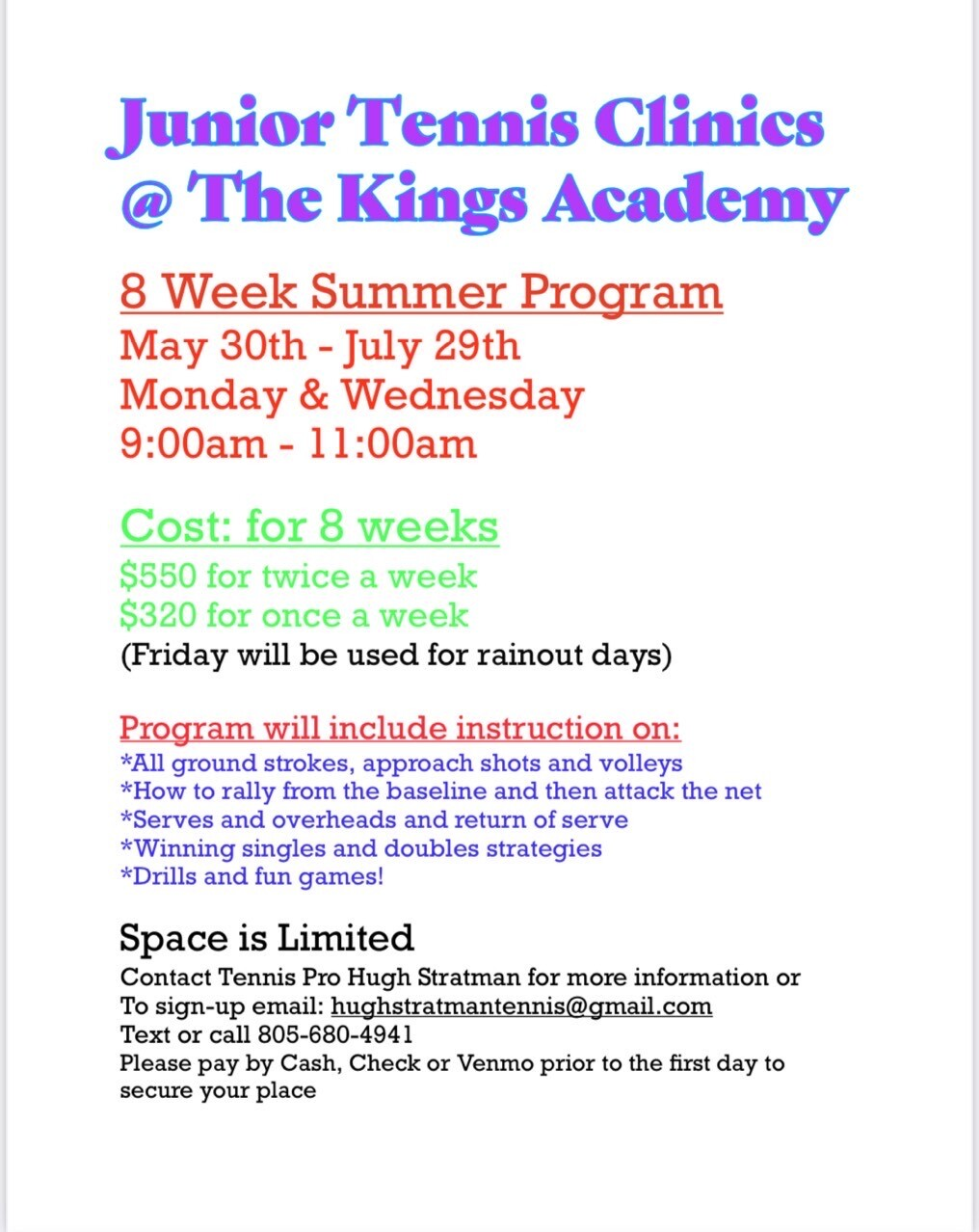# Jr. Tennis Clinics

## 8 week summer program

What: Tennis Pro Hugh Stratman will be offering an eight week tennis clinic.

Who: Middle and High school students

When: Mondays and Wednesdays from 9:00 a.m. to 11:00 a.m. starting May 30th through July 29th.

Where: TKA Tennis Courts

Cost (for 8 weeks): \$550 for twice a week
\$320 for once a week

How to sign up: Contact Hugh Stratman through text, phone call, or email at the contact information listed below:
Phone: 805-680-4941
Email: hughstratmantennis@gmail.com

 table div table+table+table+table+table+table+table+table+table+table+table+table+table+table+table+table+table+table+table+table+table+table+table+table+table+table+table+table+table+table+table+table+table+table+table+table+table+table+table+table+table+table div table{width:100%;padding:0}table div table+table+table+table+table+table+table+table+table+table+table+table+table+table+table+table+table+table+table+table+table+table+table+table+table+table+table+table+table+table+table+table+table+table+table+table+table+table+table+table+table+table div table img{width:96.23%;padding:0;float:none}table div table+table+table+table+table+table+table+table+table+table+table+table+table+table+table+table+table+table+table+table+table+table+table+table+table+table+table+table+table+table+table+table+table+table+table+table+table+table+table+table+table+table div table td{width:100%;padding:0 1.88% 18px}/* styles */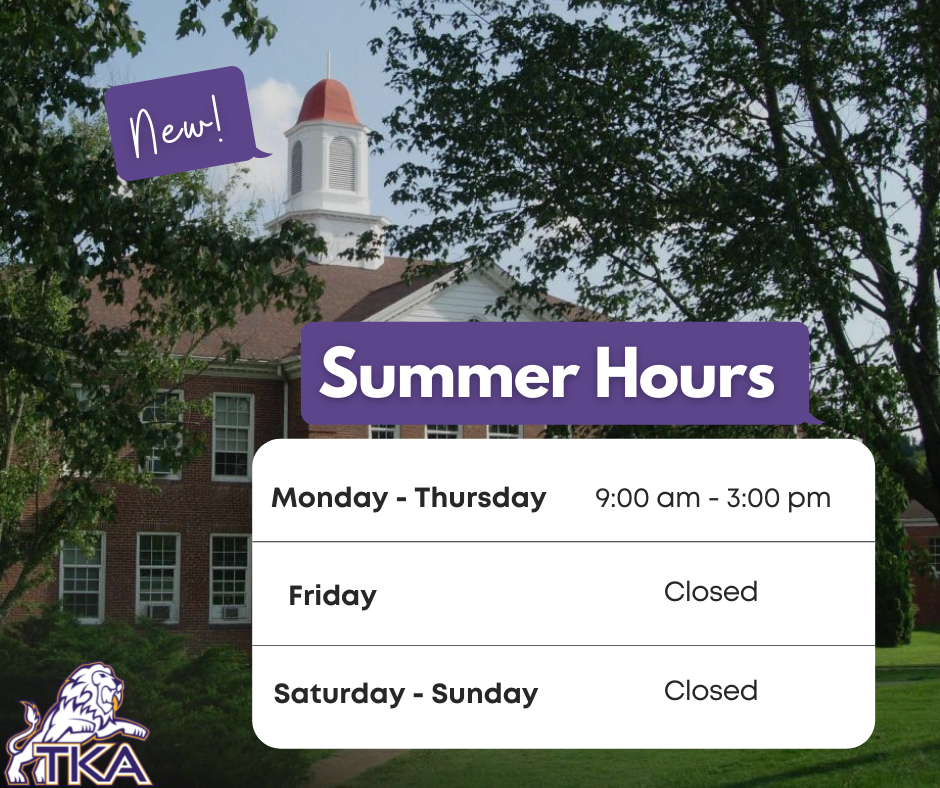# Summer 2022

This summer the school offices (Anderson and Ogle) will be open Monday - Thursday from 9:00 a.m. to 3:00 p.m. The offices will be closed Friday through Sunday.

 table div table+table+table+table+table+table+table+table+table+table+table+table+table+table+table+table+table+table+table+table+table+table+table+table+table+table+table+table+table+table+table+table+table+table+table+table+table+table+table+table+table+table+table+table div table{width:100%;padding:0}table div table+table+table+table+table+table+table+table+table+table+table+table+table+table+table+table+table+table+table+table+table+table+table+table+table+table+table+table+table+table+table+table+table+table+table+table+table+table+table+table+table+table+table+table div table img{width:96.23%;padding:0;float:none}table div table+table+table+table+table+table+table+table+table+table+table+table+table+table+table+table+table+table+table+table+table+table+table+table+table+table+table+table+table+table+table+table+table+table+table+table+table+table+table+table+table+table+table+table div table td{width:100%;padding:0 1.88% 18px}/* styles */# Spring Sport Highlights# Tennis

The girls won in 4 straight courts defeating Silverdale and are headed to substate!

They will play in the East Region seeding playoff this Thursday and Friday at Grace.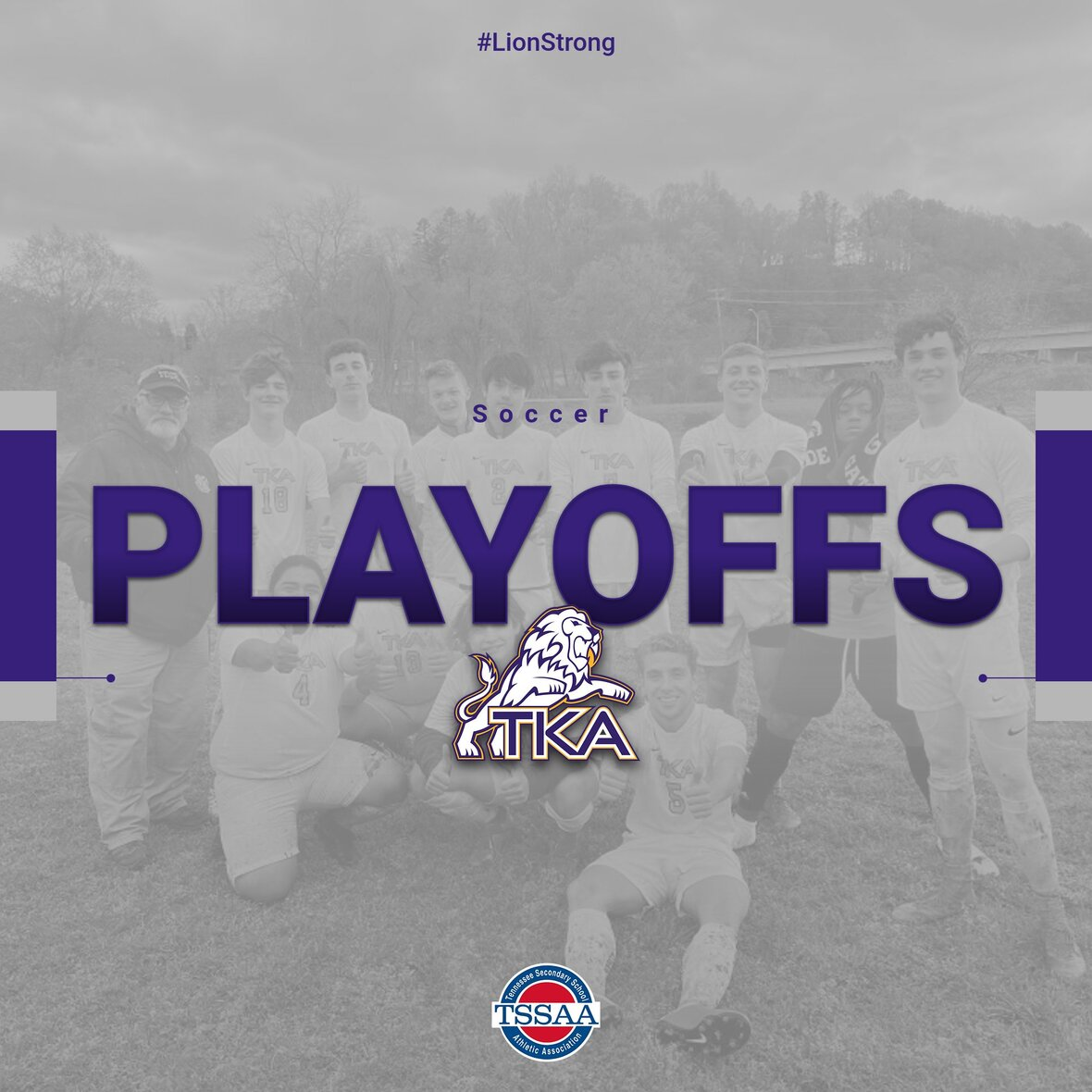# Soccer

## Middle School Soccer

Middle School Soccer will play in the championship game on Monday, May 16.

## Varsity High School Soccer

Varsity Soccer will play on Thursday, May 12, and Tuesday, May 17.

Times and opponents are TBD as results of the playoff games. Once times are made available, those will be communicated as we hope to see everyone out cheering on both of our soccer teams!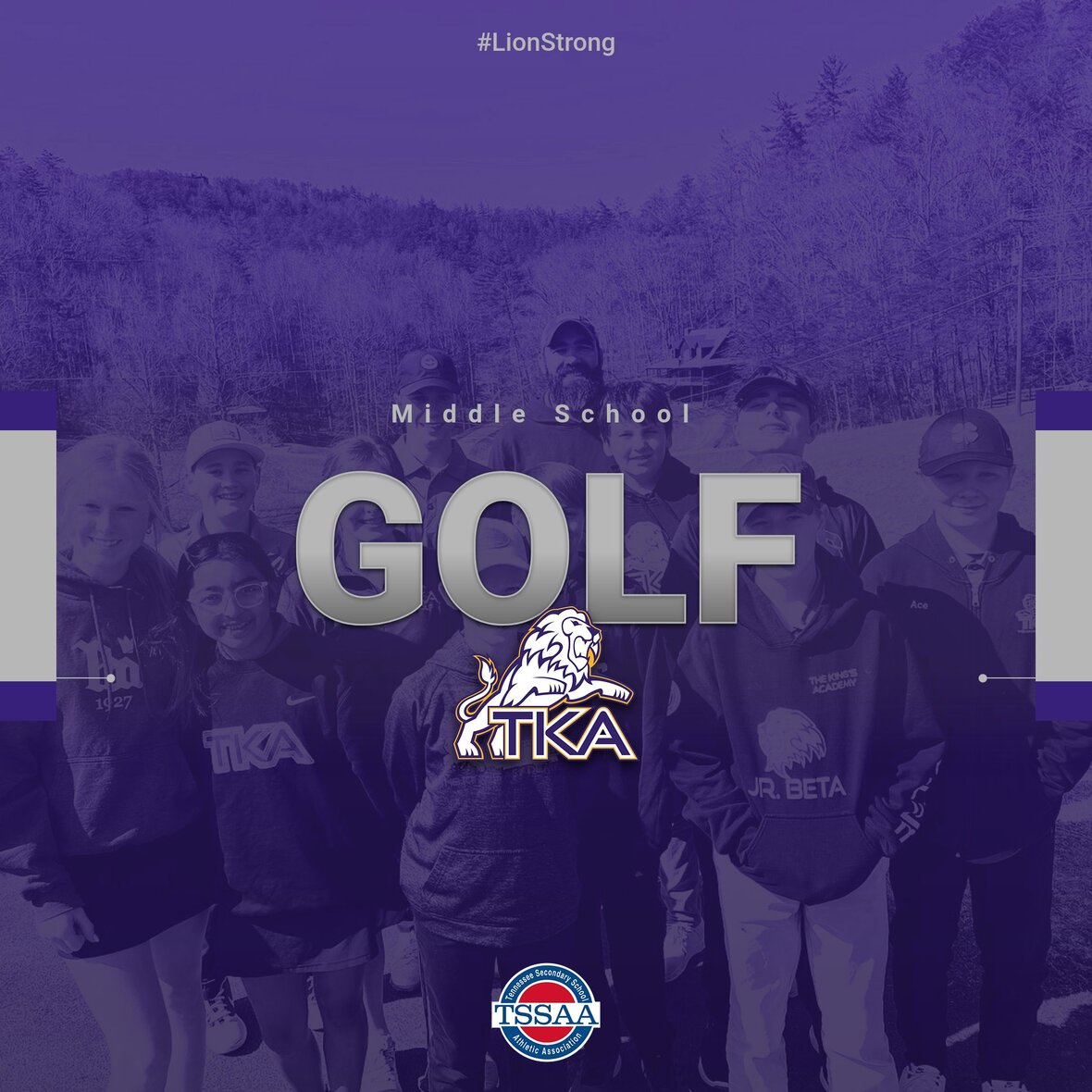# Middle School Golf

Three of our Middle School golfers advanced to the Regional Golf Tournament!

The TKA boys team will be represented by Brody Byers, and the The Lady Lions will be represented by Maggie McKinney & Delana Pritchard. We are proud of how hard all of our golf team has worked and represented TKA on and off the course!

The tournament will be on May 12th starting at 8:00am at Oak Ridge CC.

 table div table+table+table+table+table+table+table+table+table+table+table+table+table+table+table+table+table+table+table+table+table+table+table+table+table+table+table+table+table+table+table+table+table+table+table+table+table+table+table+table+table+table+table+table+table+table+table+table+table div table{width:100%;padding:0}table div table+table+table+table+table+table+table+table+table+table+table+table+table+table+table+table+table+table+table+table+table+table+table+table+table+table+table+table+table+table+table+table+table+table+table+table+table+table+table+table+table+table+table+table+table+table+table+table+table div table img{width:96.23%;padding:0;float:none}table div table+table+table+table+table+table+table+table+table+table+table+table+table+table+table+table+table+table+table+table+table+table+table+table+table+table+table+table+table+table+table+table+table+table+table+table+table+table+table+table+table+table+table+table+table+table+table+table+table div table td{width:100%;padding:0 1.88% 18px}/* styles */# Upcoming Sports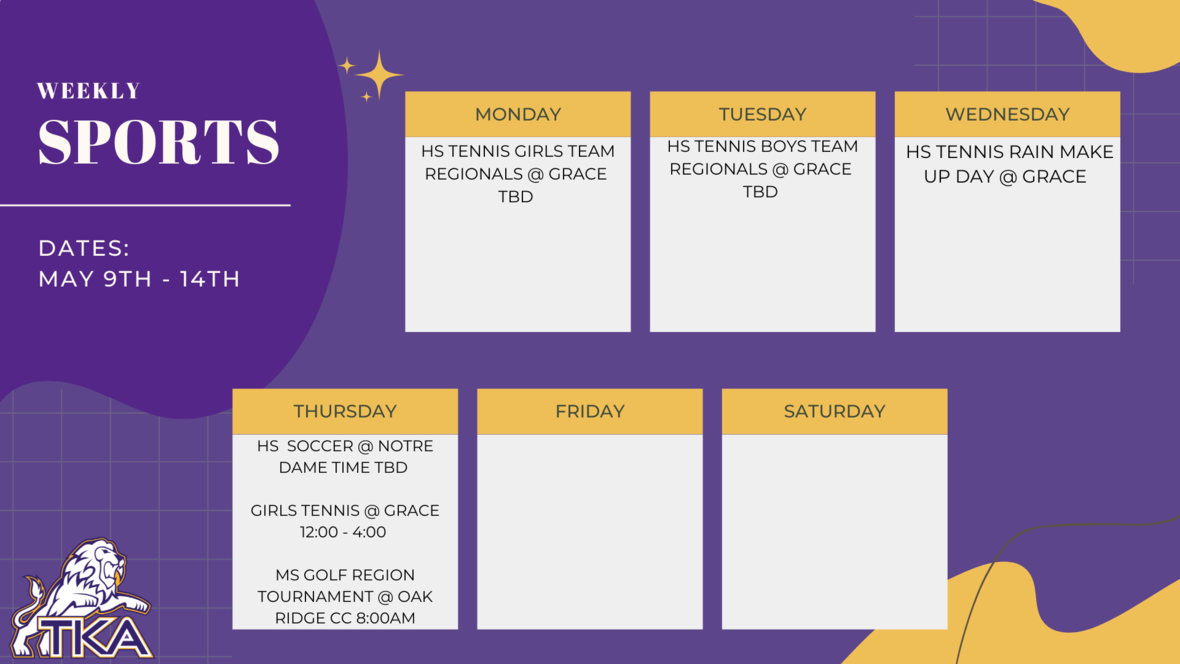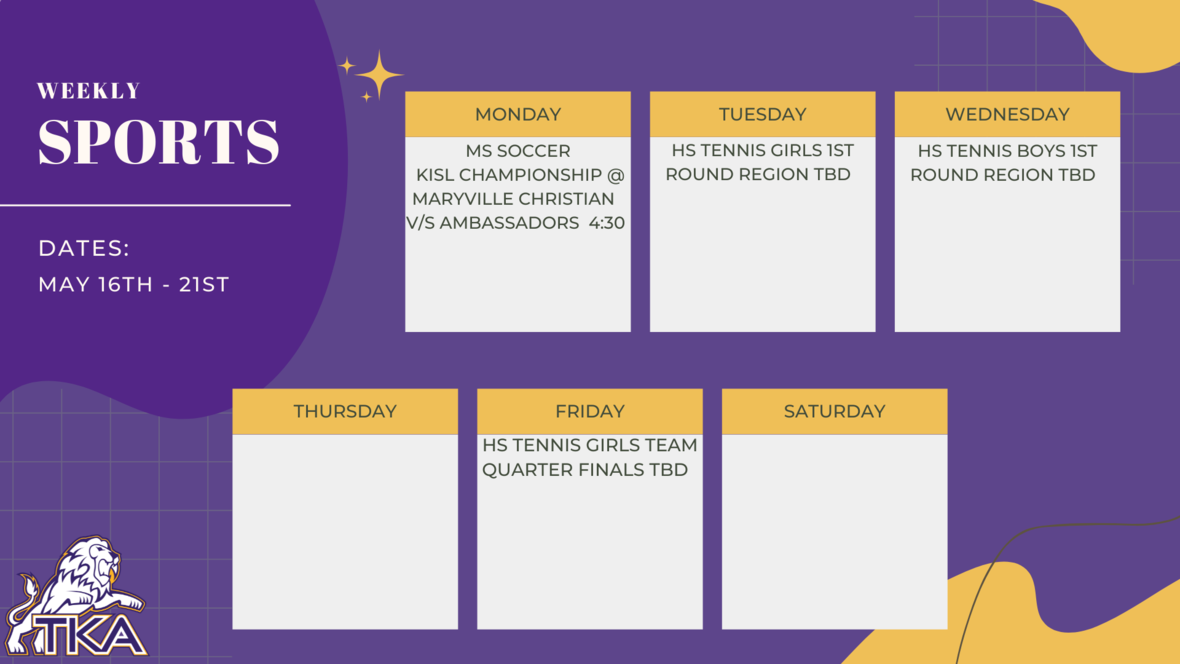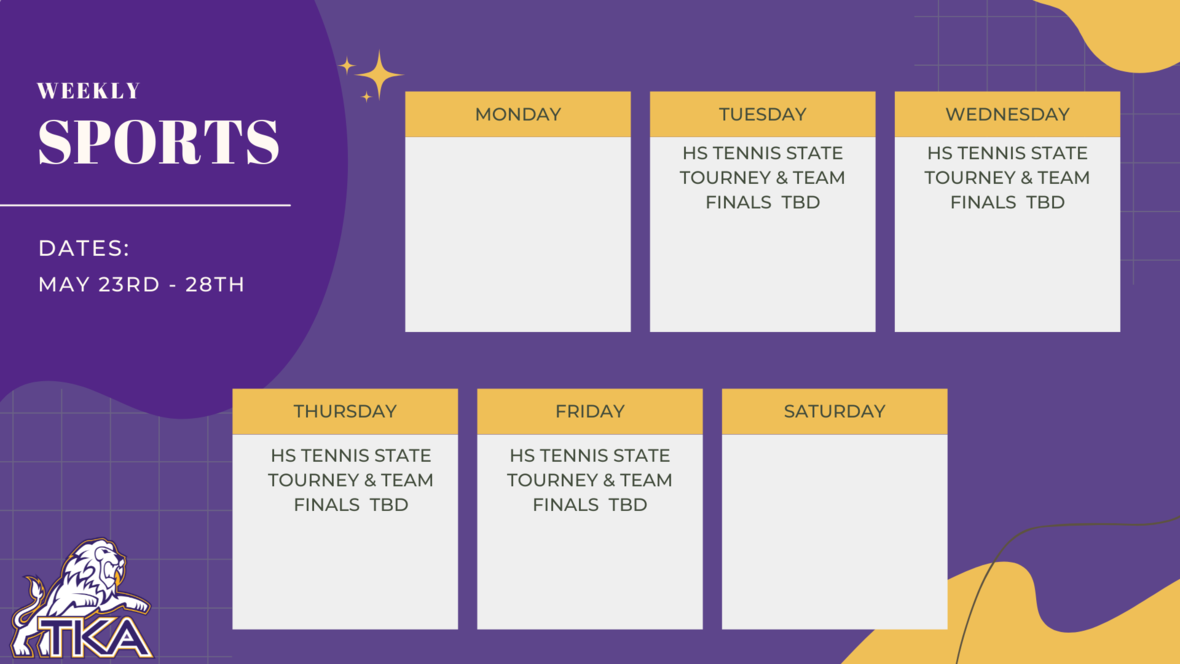table div table+table+table+table+table+table+table+table+table+table+table+table+table+table+table+table+table+table+table+table+table+table+table+table+table+table+table+table+table+table+table+table+table+table+table+table+table+table+table+table+table+table+table+table+table+table+table+table+table+table+table+table+table+table div table{width:100%;padding:0}table div table+table+table+table+table+table+table+table+table+table+table+table+table+table+table+table+table+table+table+table+table+table+table+table+table+table+table+table+table+table+table+table+table+table+table+table+table+table+table+table+table+table+table+table+table+table+table+table+table+table+table+table+table+table div table img{width:96.23%;padding:0;float:none}table div table+table+table+table+table+table+table+table+table+table+table+table+table+table+table+table+table+table+table+table+table+table+table+table+table+table+table+table+table+table+table+table+table+table+table+table+table+table+table+table+table+table+table+table+table+table+table+table+table+table+table+table+table+table div table td{width:100%;padding:0 1.88% 18px}/* styles */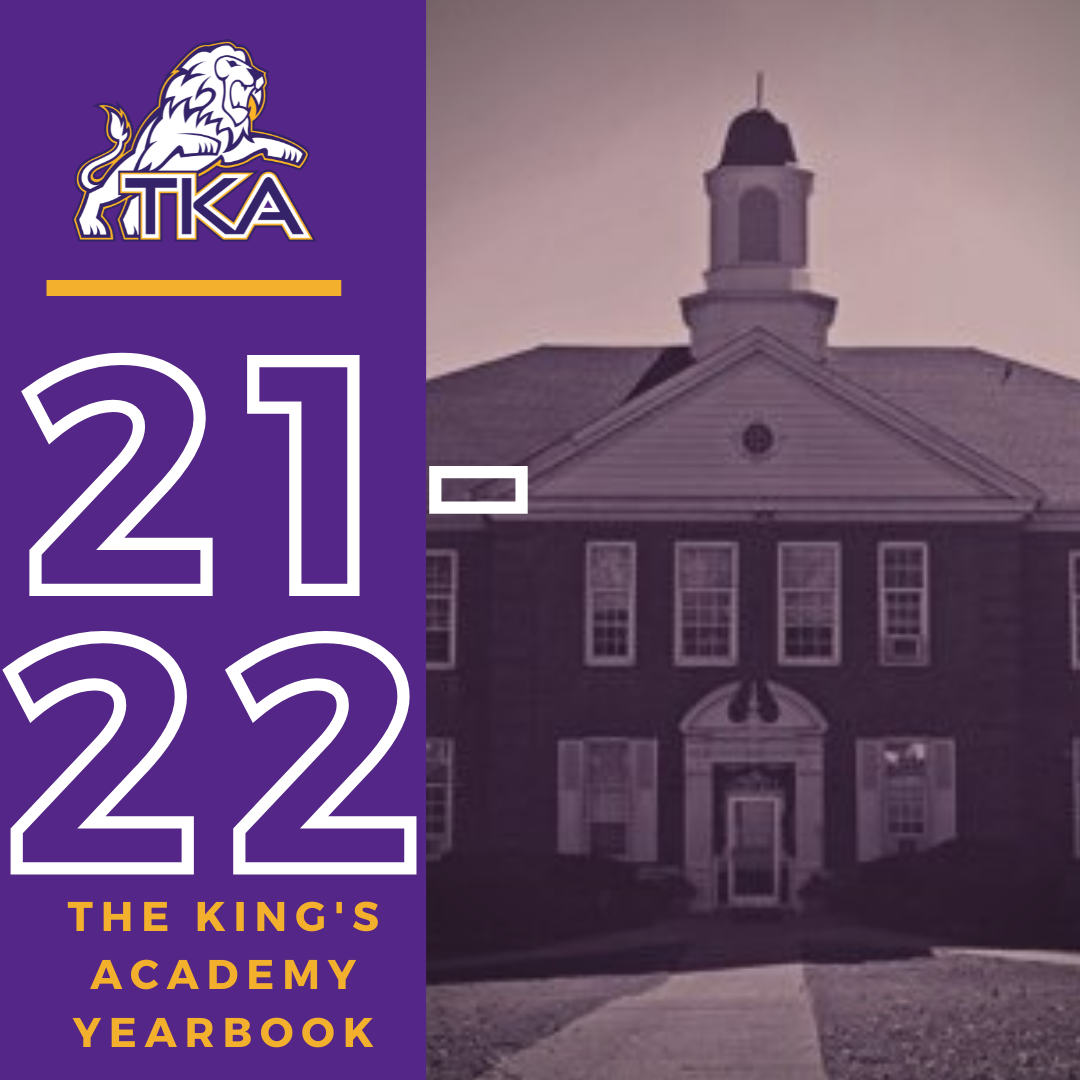# 2021-2022 Yearbook

The price for the 2021-2022 TKA yearbook is \$55. All grades, K3-12 will have the same yearbook. The slight price increase is because we are only going to have one larger all-school book.

All yearbook orders can be placed online--here is the link.Do you have a class of 2022 senior in your household?

Don't miss out on the opportunity to purchase a Senior Tribute for the 2021-2022 yearbook!

Senior Tributes are available for purchase at the bottom of the link listed here.For any questions regarding yearbooks, email Mr. Josh Smith at jsmith@thekingsacademy.net.

 table div table+table+table+table+table+table+table+table+table+table+table+table+table+table+table+table+table+table+table+table+table+table+table+table+table+table+table+table+table+table+table+table+table+table+table+table+table+table+table+table+table+table+table+table+table+table+table+table+table+table+table+table+table+table+table+table+table+table div table{width:100%;padding:0}table div table+table+table+table+table+table+table+table+table+table+table+table+table+table+table+table+table+table+table+table+table+table+table+table+table+table+table+table+table+table+table+table+table+table+table+table+table+table+table+table+table+table+table+table+table+table+table+table+table+table+table+table+table+table+table+table+table+table div table img{width:96.23%;padding:0;float:none}table div table+table+table+table+table+table+table+table+table+table+table+table+table+table+table+table+table+table+table+table+table+table+table+table+table+table+table+table+table+table+table+table+table+table+table+table+table+table+table+table+table+table+table+table+table+table+table+table+table+table+table+table+table+table+table+table+table+table div table td{width:100%;padding:0 1.88% 18px}/* styles */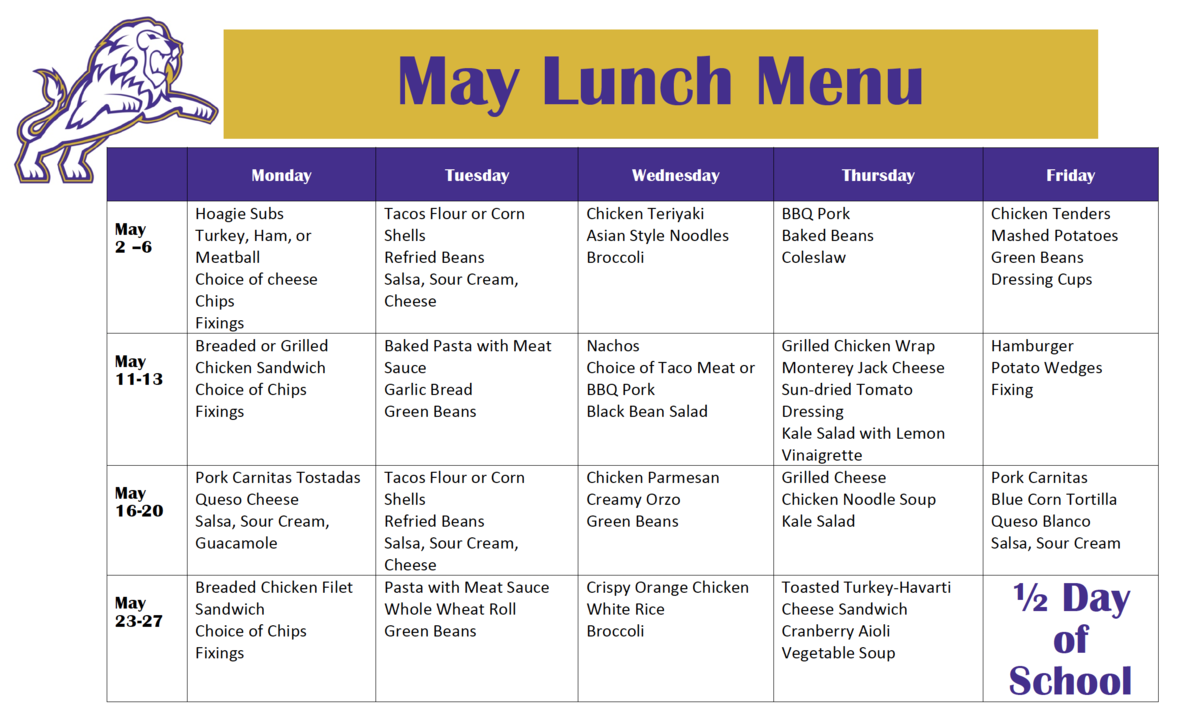table div table+table+table+table+table+table+table+table+table+table+table+table+table+table+table+table+table+table+table+table+table+table+table+table+table+table+table+table+table+table+table+table+table+table+table+table+table+table+table+table+table+table+table+table+table+table+table+table+table+table+table+table+table+table+table+table+table+table+table+table div table{width:100%;padding:0}table div table+table+table+table+table+table+table+table+table+table+table+table+table+table+table+table+table+table+table+table+table+table+table+table+table+table+table+table+table+table+table+table+table+table+table+table+table+table+table+table+table+table+table+table+table+table+table+table+table+table+table+table+table+table+table+table+table+table+table+table div table img{width:96.23%;padding:0;float:none}table div table+table+table+table+table+table+table+table+table+table+table+table+table+table+table+table+table+table+table+table+table+table+table+table+table+table+table+table+table+table+table+table+table+table+table+table+table+table+table+table+table+table+table+table+table+table+table+table+table+table+table+table+table+table+table+table+table+table+table+table div table td{width:100%;padding:0 1.88% 18px}/* styles *//* styles */
 table div table+table+table+table+table+table+table+table+table+table+table+table+table+table+table+table+table+table+table+table+table+table+table+table+table+table+table+table+table+table+table+table+table+table+table+table+table+table+table+table+table+table+table+table+table+table+table+table+table+table+table+table+table+table+table+table+table+table+table+table+table+table+table div table{width:100%;padding:0}table div table+table+table+table+table+table+table+table+table+table+table+table+table+table+table+table+table+table+table+table+table+table+table+table+table+table+table+table+table+table+table+table+table+table+table+table+table+table+table+table+table+table+table+table+table+table+table+table+table+table+table+table+table+table+table+table+table+table+table+table+table+table+table div table img{width:96.23%;padding:0;float:none}table div table+table+table+table+table+table+table+table+table+table+table+table+table+table+table+table+table+table+table+table+table+table+table+table+table+table+table+table+table+table+table+table+table+table+table+table+table+table+table+table+table+table+table+table+table+table+table+table+table+table+table+table+table+table+table+table+table+table+table+table+table+table+table div table td{width:100%;padding:0 1.88% 18px}/* styles */# Covid-19 Procedures

While we are excited to offer in-person education, we must maintain a safe learning environment for all students, faculty/staff, and additional stakeholders.

To do so, we will be working closely with the Sevier County Health Department regarding Covid-19 protocols.

 table div table+table+table+table+table+table+table+table+table+table+table+table+table+table+table+table+table+table+table+table+table+table+table+table+table+table+table+table+table+table+table+table+table+table+table+table+table+table+table+table+table+table+table+table+table+table+table+table+table+table+table+table+table+table+table+table+table+table+table+table+table+table+table+table+table div table{width:100%;padding:0}table div table+table+table+table+table+table+table+table+table+table+table+table+table+table+table+table+table+table+table+table+table+table+table+table+table+table+table+table+table+table+table+table+table+table+table+table+table+table+table+table+table+table+table+table+table+table+table+table+table+table+table+table+table+table+table+table+table+table+table+table+table+table+table+table+table div table img{width:96.23%;padding:0;float:none}table div table+table+table+table+table+table+table+table+table+table+table+table+table+table+table+table+table+table+table+table+table+table+table+table+table+table+table+table+table+table+table+table+table+table+table+table+table+table+table+table+table+table+table+table+table+table+table+table+table+table+table+table+table+table+table+table+table+table+table+table+table+table+table+table+table div table td{width:100%;padding:0 1.88% 18px}/* styles */table div table+table+table+table+table+table+table+table+table+table+table+table+table+table+table+table+table+table+table+table+table+table+table+table+table+table+table+table+table+table+table+table+table+table+table+table+table+table+table+table+table+table+table+table+table+table+table+table+table+table+table+table+table+table+table+table+table+table+table+table+table+table+table+table+table+table+table div table{width:100%;padding:0}table div table+table+table+table+table+table+table+table+table+table+table+table+table+table+table+table+table+table+table+table+table+table+table+table+table+table+table+table+table+table+table+table+table+table+table+table+table+table+table+table+table+table+table+table+table+table+table+table+table+table+table+table+table+table+table+table+table+table+table+table+table+table+table+table+table+table+table div table img{width:96.23%;padding:0;float:none}table div table+table+table+table+table+table+table+table+table+table+table+table+table+table+table+table+table+table+table+table+table+table+table+table+table+table+table+table+table+table+table+table+table+table+table+table+table+table+table+table+table+table+table+table+table+table+table+table+table+table+table+table+table+table+table+table+table+table+table+table+table+table+table+table+table+table+table div table td{width:100%;padding:0 1.88% 18px}/* styles */